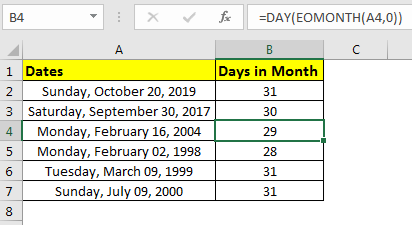# How to Count Days in Month in ExcelWell, this is one can be done manually but when you work on excel you want automation as much as possible. In that case, for getting days in given month, we should use excel formulas. In this article, we will learn how to get number of days in given month.

Generic Formula

=DAY(EOMONTH(date,0))

Date: the number of days will be calculated of this date’s month.

Example: Get number of days in month of sum random dates.
Here, we have this list of sum random dates. We need to get number of days of respective months. If you have to tell me the number of days in each month, you will take significant time to tell months with 31 days and 30 days, and may forget to check the leap year. Excel will not do these mistakes.

Write this formula in cell B2 and drag it down.

=DAY(EOMONTH(A2,0))

This can be used to calculate salaries and to know workdays.
How it works?
The EOMONTH function of excel returns the serial number of last date of month of given date. The DAY function returns the Day from last date of month. Together they provide us number of days in month of given date.

So yeah guys, this how you can get number of days of a month in excel. Let me know if you have any doubt or query regarding this article or another excel topic. The comments section is open for you. We will be happy to help you.

Popular Articles

50 Excel Shortcut to Increase Your Productivity: Get faster at your task. These 50 shortcuts will make you work even faster on Excel.

How to use the VLOOKUP Function in Excel: This is one of the most used and popular functions of excel that is used to lookup value from different ranges and sheets.

How to use the COUNTIF function in Excel: Count values with conditions using this amazing function. You don't need to filter your data to count specific values. Countif function is essential to prepare your dashboard.

How to use the SUMIF Function in Excel: This is another dashboard essential function. This helps you sum up values on specific conditions.

1.I would like a formula to calculate days remaining, but differently. I am a Canadian that is only allowed to stay for 182 days in the US. I would like to be able to count from Jan. 1, and be shown how many days I have left after I remove May through Nov.. Know what I mean .

•